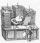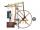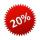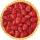Arble bag

A marble bag sold by Rachel's Marble Company contains 5 orange marbles for every 6 green marbles. If a bag has 35 orange marbles, how many green marbles does it contain?

Result

g =  42

Solution:Leave us a comment of this math problem and its solution (i.e. if it is still somewhat unclear...):Be the first to comment!Next similar math problems:

1. BureaucracyThe office was attended by 23 people. 9 of them completed the questionnaire. What is the percentage of all people?
2. Ratio v2Decrease in the ratio 12:16 number 13.2.
3. RatioIncrease in the ratio 20:4 number 18.5.
4. EfficiencyA certain device consumes 28 kW and delivers 14 kW. Find its efficiency.
5. DonutsFind how many donuts each student will receive if you share 126 donuts in a ratio of 1:5:8
6. Percentages and numbersHow many percent is number 426 greater than number 71?
7. 1.5 divided1.5 divided by 1 = w divided by 4
8. Two numbersFind two numbers whose difference and ratio is 2.
9. Strange xFor what x is true ??
10. Sales offGoods is worth € 70 and the price of goods fell two weeks in a row by 10%. How many % decreased overall?
11. Apples 2James has 13 apples. He has 30 percent more apples than Sam. How many apples has Sam?
12. Percents - easyHow many percent is 432 out of 434?
13. TVsProduction of television sets increased from 3,500 units to 4,200 units. Calculate the percentage of production increase.
14. Conference148 is the total number of employees. The conference was attended by 22 employees. How much is it in percent?
15. MO 2016 Numerical axisCat's school use a special numerical axis. The distance between the numbers 1 and 2 is 1 cm, the distance between the numbers 2 and 3 is 3 cm, between the numbers 3 and 4 is 5 cm and so on, the distance between the next pair of natural numbers is always in
16. Down syndromeDown syndrome is one of the serious diseases caused by a gene mutation. Down syndrome occurs in approximately every 550-born child. Express the incidence of Down's syndrome in newborns at per mille.
17. NumberWhat number is 20 % smaller than the number 198?